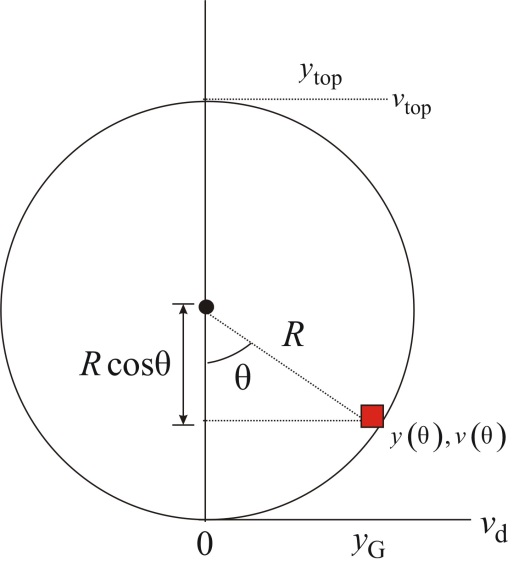Problem

# A very slippery ice cube slides in a vertical plane around the inside of a smooth, 20-cm-d...

A very slippery ice cube slides in a vertical plane around the inside of a smooth, 20-cm-diameter horizontal pipe. The ice cube’s speed at the bottom of the circle is 3.0 m/s.

a. What is the ice cube’s speed at the top?

b. Find an algebraic expression for the ice cube’s speed when it is at angle 9, where the angle is measured counterclockwise from the bottom of the circle. Your expression should give 3.0 m/s for θ = 0° and your answer to part a for θ = 180°.

#### Step-by-Step Solution

Solution 1

The initial kinetic energy of the ice cube is,

$$K_{\mathrm{i}}=\frac{1}{2} m v_{i}^{2}$$

The final kinetic energy of the ice cube is,

$$K_{r}=\frac{1}{2} m v_{f}^{2}$$

Here, $$m$$ is the mass of the ice cube, $$v_{f}$$ is the final velocity, and $$v_{i}$$ is the initial velocity of the ice cube.

The initial potential energy of the ice cube is,

$$U_{i}=m g y_{i}$$

Here, $$g$$ is the acceleration due to gravity and $$y_{i}$$ is the initial height.

The final potential energy of the ice cube is,

$$U_{\mathrm{r}}=m g y_{\mathrm{r}}$$

Here, $$y_{\mathrm{f}}$$ is the final height of the ice cube.

Substitute $$\frac{1}{2} m v_{f}^{2}$$ for $$K_{f}, \frac{1}{2} m v_{i}^{2}$$ for $$K_{i}, m g y_{f}$$ for $$U_{f}$$, and $$m g y_{i}$$ for $$U_{i}$$ in the equation $$K_{\mathrm{f}}+U_{\mathrm{f}}=K_{\mathrm{i}}+U_{\mathrm{i}}$$ and solve for $$v_{\mathrm{f}}$$.

\begin{aligned} \frac{1}{2} m v_{\mathrm{f}}^{2}+m g y_{\mathrm{f}} &=\frac{1}{2} m v_{\mathrm{i}}^{2}+m g y_{\mathrm{i}} \\ \frac{1}{2} m v_{\mathrm{f}}^{2} &=\frac{1}{2} m v_{\mathrm{i}}^{2}+m g y_{\mathrm{i}}-m g y_{\mathrm{f}} \\ v_{\mathrm{r}}^{2} &=v_{i}^{2}+2 g y_{\mathrm{i}}-2 g y_{\mathrm{r}} \\ v_{\mathrm{f}} &=\sqrt{v_{\mathrm{i}}^{2}+2 g y_{\mathrm{i}}-2 g y_{\mathrm{f}}} \end{aligned}

Convert the units of $$y_{i}$$ from $$\mathrm{cm}$$ to $$\mathrm{m}$$.

\begin{aligned} y_{i} &=(20 \mathrm{~cm})\left(\frac{1 \mathrm{~m}}{100 \mathrm{~cm}}\right) \\ &=0.2 \mathrm{~m} \end{aligned}

Substitute $$3.0 \mathrm{~m} / \mathrm{s}$$ for $$v_{f}, 9.80 \mathrm{~m} / \mathrm{s}^{2}$$ for $$g, 0 \mathrm{~m}$$ for $$y_{r}$$, and $$0.2 \mathrm{~m}$$ for $$y_{i}$$ in the equation $$v_{\mathrm{f}}=\sqrt{v_{i}^{2}+2 g y_{i}-2 g y_{\mathrm{r}}}$$ and solve for $$v_{\mathrm{f}}$$.

\begin{aligned} v_{\mathrm{i}} &=\sqrt{(3.0 \mathrm{~m} / \mathrm{s})^{2}+2\left(9.80 \mathrm{~m} / \mathrm{s}^{2}\right)(0 \mathrm{~m}-0.2 \mathrm{~m})} \\ &=2.25 \mathrm{~m} / \mathrm{s} \end{aligned}

Round off to two significant figures, the speed of ice cube at the top is $$2.3 \mathrm{~m} / \mathrm{s}$$.

(b)

Express the law of energy conservation of energy as a function of $$\theta$$.

$$\begin{gathered} K(\theta)+U_{\mathrm{g}}(\theta)=K_{\text {Botoom }}+U_{\text {g.Bottom }} \\ \frac{1}{2} m v^{2}(\theta)+m g y(\theta)=\frac{1}{2} m v_{\text {Botion }}^{2}+m g y_{\text {Bontom }} \end{gathered}$$

Here, $$v_{\text {nonson }}$$ is the velocity at bottom of the pipe, and $$y_{\text {hinnor }}$$ is the height at the bottom of pipe. Substitute 0 for $$y_{\text {Borrom }}$$ in the above equation and rewrite to solve for $$v(\theta)$$.

\begin{aligned} \frac{1}{2} m v^{2}(\theta)+m g y(\theta) &=\frac{1}{2} m v_{\text {Bonown }}^{2}+0 \\ m v^{2}(\theta)+2 m g y(\theta) &=m v_{\text {Bottem }}^{2} \\ v^{2}(\theta) &=v_{\text {Boflom }}^{2}-2 g y(\theta) \\ v(\theta) &=\sqrt{v_{\text {Boteon }}^{2}-2 g y(\theta)} \end{aligned}

Below figure shows the arrangement office cube in the vertical circular loop.Use the above figure; at an arbitrary angle $$\theta$$ measured counter clockwise from the bottom of the circle is as follows:

\begin{aligned} y(\theta) &=R-R \cos \theta \\ &=R(1-\cos \theta) \end{aligned}

Substitute $$R-R \cos \theta$$ for $$y(\theta)$$ in the equation $$v(\theta)=\sqrt{v_{\text {Botom }}^{2}-2 g y(\theta)}$$ and solve for $$v(\theta)$$.

$$v(\theta)=\sqrt{v_{\text {Botom }}^{2}-2 g R(1-\cos \theta)}$$

Substitute $$3.0 \mathrm{~m} / \mathrm{s}$$ for $$v_{\text {inonsm }}+10 \mathrm{~cm}$$ for $$R$$, and $$9.8 \mathrm{~m} / \mathrm{s}^{2}$$ for $$g$$.

\begin{aligned} v(\theta) &=\sqrt{(3.0 \mathrm{~m} / \mathrm{s})^{2}-2\left(9.8 \mathrm{~m} / \mathrm{s}^{2}\right)\left(10 \mathrm{~cm}\left(\frac{1 \mathrm{~m}}{100 \mathrm{~cm}}\right)\right)(1-\cos \theta)} \\ &=\sqrt{9-1.96(1-\cos \theta)} \mathrm{m} / \mathrm{s} \end{aligned}

Hence, the expression for ice cube's speed when it is at angle $$\theta$$ is

$$v(\theta)=\sqrt{9-1.96(1-\cos \theta)} \mathrm{m} / \mathrm{s}$$